Next: Boundary Condition Designation. Up: GF Numbering System. Previous: GF Numbering System.

## Coordinate System Designation.

The GF Library includes three coordinate systems: rectangular, cylindrical, and spherical. The numbering system has letters to denote coordinates in each coordinate system, and one letter is needed to indicate each coordinate present. Thus, a one-dimensional body designation contains one letter; a 2-D body designation contains two letters, and, a 3-D body designation contains three letters.

Rectangular coordinate system (x, y, z). Symbol X is used to denote the x-coordinate, Y denotes the y-coordinate, and Z denotes the z-coordinate. Example: a 1-D plate with type 2 boundaries is designated X22.

Cylindrical coordinate system (r,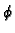, x). Symbol R is used to denote the r-coordinate, symbol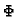denotes angle( 0 << 2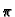), and symbol X denotes the axial x-coordinate. Example: 1-D radial heat transfer in a hollow cylindrical shell with both surfaces convectively heated is designated R33.

Spherical (polar) coordinate system (r,,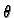). Symbol RS is used to denote the spherical r-coordinate, symboldenotes azimuthal angle( 0 << 2), and symbol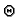denotes the polar angle( 0 <<). Example: 1-D radial heat transfer in a hollow sphere with type 1 boundary conditions is designated RS11.Next: Boundary Condition Designation. Up: GF Numbering System. Previous: GF Numbering System.
Kevin D. Cole
2003-07-21